Warning

This documents an unmaintained version of NetworkX. Please upgrade to a maintained version and see the current NetworkX documentation.

# betweenness_centrality¶

betweenness_centrality(G, k=None, normalized=True, weight=None, endpoints=False, seed=None)[source]

Compute the shortest-path betweenness centrality for nodes.

Betweenness centrality of a node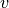is the sum of the fraction of all-pairs shortest paths that pass through: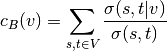where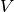is the set of nodes,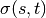is the number of shortest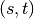-paths, and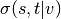is the number of those paths passing through some nodeother than. If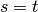,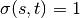, and if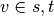,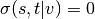.

Parameters: G (graph) – A NetworkX graph k (int, optional (default=None)) – If k is not None use k node samples to estimate betweenness. The value of k <= n where n is the number of nodes in the graph. Higher values give better approximation. normalized (bool, optional) – If True the betweenness values are normalized by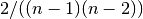for graphs, and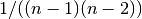for directed graphs where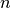is the number of nodes in G. weight (None or string, optional) – If None, all edge weights are considered equal. Otherwise holds the name of the edge attribute used as weight. endpoints (bool, optional) – If True include the endpoints in the shortest path counts. nodes – Dictionary of nodes with betweenness centrality as the value. dictionary

Notes

The algorithm is from Ulrik Brandes . See  for the original first published version and  for details on algorithms for variations and related metrics.

For approximate betweenness calculations set k=#samples to use k nodes (“pivots”) to estimate the betweenness values. For an estimate of the number of pivots needed see .

For weighted graphs the edge weights must be greater than zero. Zero edge weights can produce an infinite number of equal length paths between pairs of nodes.

References

  Ulrik Brandes: A Faster Algorithm for Betweenness Centrality. Journal of Mathematical Sociology 25(2):163-177, 2001. http://www.inf.uni-konstanz.de/algo/publications/b-fabc-01.pdf
  (1, 2) Ulrik Brandes: On Variants of Shortest-Path Betweenness Centrality and their Generic Computation. Social Networks 30(2):136-145, 2008. http://www.inf.uni-konstanz.de/algo/publications/b-vspbc-08.pdf
  Ulrik Brandes and Christian Pich: Centrality Estimation in Large Networks. International Journal of Bifurcation and Chaos 17(7):2303-2318, 2007. http://www.inf.uni-konstanz.de/algo/publications/bp-celn-06.pdf
  Linton C. Freeman: A set of measures of centrality based on betweenness. Sociometry 40: 35–41, 1977 http://moreno.ss.uci.edu/23.pdf